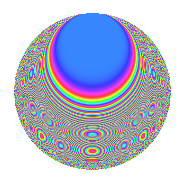# Properties

 Label 430.2.lLevel 430 Weight 2 Character orbit l Rep. character $$\chi_{430}(7,\cdot)$$ Character field $$\Q(\zeta_{12})$$ Dimension 88 Newform subspaces 1 Sturm bound 132 Trace bound 0

# Related objects

## Defining parameters

 Level: $$N$$ = $$430 = 2 \cdot 5 \cdot 43$$ Weight: $$k$$ = $$2$$ Character orbit: $$[\chi]$$ = 430.l (of order $$12$$ and degree $$4$$) Character conductor: $$\operatorname{cond}(\chi)$$ = $$215$$ Character field: $$\Q(\zeta_{12})$$ Newform subspaces: $$1$$ Sturm bound: $$132$$ Trace bound: $$0$$

## Dimensions

The following table gives the dimensions of various subspaces of $$M_{2}(430, [\chi])$$.

Total New Old
Modular forms 280 88 192
Cusp forms 248 88 160
Eisenstein series 32 0 32

## Trace form

 $$88q + 4q^{6} + 12q^{7} + O(q^{10})$$ $$88q + 4q^{6} + 12q^{7} + 16q^{13} - 88q^{16} + 4q^{17} - 16q^{21} + 12q^{23} - 8q^{25} + 12q^{28} - 36q^{30} - 40q^{31} + 12q^{33} - 40q^{35} - 40q^{36} + 16q^{38} - 56q^{41} - 56q^{43} + 24q^{46} + 72q^{47} + 24q^{50} - 16q^{52} + 20q^{53} + 24q^{55} - 8q^{56} + 20q^{57} + 8q^{60} + 72q^{61} + 36q^{62} - 40q^{66} - 16q^{67} + 4q^{68} + 24q^{71} + 60q^{73} - 48q^{76} - 48q^{77} + 40q^{78} + 28q^{81} + 20q^{86} - 64q^{87} - 8q^{90} + 48q^{91} - 12q^{92} - 108q^{93} + 4q^{95} - 4q^{96} - 48q^{97} - 96q^{98} + O(q^{100})$$

## Decomposition of $$S_{2}^{\mathrm{new}}(430, [\chi])$$ into newform subspaces

Label Dim. $$A$$ Field CM Traces $q$-expansion
$$a_2$$ $$a_3$$ $$a_5$$ $$a_7$$
430.2.l.a $$88$$ $$3.434$$ None $$0$$ $$0$$ $$0$$ $$12$$

## Decomposition of $$S_{2}^{\mathrm{old}}(430, [\chi])$$ into lower level spaces

$$S_{2}^{\mathrm{old}}(430, [\chi]) \cong$$ $$S_{2}^{\mathrm{new}}(215, [\chi])$$$$^{\oplus 2}$$

## Hecke Characteristic Polynomials

There are no characteristic polynomials of Hecke operators in the database